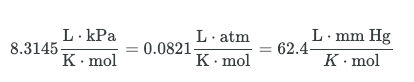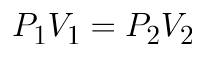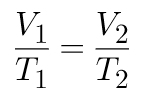MCAT Content / Gas Phase / Ideal Gas

### Ideal Gas

Topic: Gas Phase

An ideal gas exhibits no attractive forces between particles.

All gases are modeled on the assumptions put forth by the Kinetic Theory of Matter, which assumes that all matter is made up of particles (i.e. atoms or molecules); there are spaces between these particles, and attractive forces become stronger as the particles converge. Particles are in constant, random motion, and they collide with one another and the walls of the container in which they are enclosed. Each particle has an inherent kinetic energy that is dependent upon temperature only.

A gas is considered ideal if its particles are so far apart that they do not exert any attractive forces upon one another. In real life, there is no such thing as a truly ideal gas, but at high temperatures and low pressures (conditions in which individual particles will be moving very quickly and be very far apart from one another so that their interaction is almost zero), gases behave close to ideally; this is why the Ideal Gas Law is such a useful approximation.

Ideal Gas Law

The Ideal Gas Equation is given by:

PV = nRT

The four variables represent four different properties of a gas:

• Pressure (P), often measured in atmospheres (atm), kilopascals (kPa), or millimeters mercury/torr (mm Hg, torr)
• Volume (V), given in liters
• Number of moles of gas (n)
• Temperature of the gas (T) measured in degrees Kelvin (K)

R is the ideal gas constant, which takes on different forms depending on which units are in use. The three most common formulations of R are given by:The ideal gas equation enables us to examine the relationship between the non-constant properties of ideal gases (n, P, V, T) as long as three of these properties remain fixed.

For the ideal gas equation, note that the product PV is directly proportional to T. This means that if the gas’ temperature remains constant, pressure or volume can increase as long as the complementary variable decreases; this also means that if the gas’ temperature changes, it may be due in part to a change in the variable of pressure or volume.

The ideal gas equation is a valuable tool that can give a very good approximation of gases at high temperatures and low pressures.

Boyles Law

Boyle’s Law (sometimes referred to as the Boyle-Mariotte Law) states that the absolute pressure and volume of a given mass of confined gas are inversely proportional, provided the temperature remains unchanged within a closed system. This can be stated mathematically as follows:The law itself can be stated as follows: for a fixed amount of an ideal gas kept at a fixed temperature, P (pressure) and V (volume) are inversely proportional—that is, when one doubles, the other is reduced by half. Remember that these relations hold true only if the number of molecules (n) and the temperature (T) are both constant.

Charles Law

Charles’ Law describes the relationship between the volume and temperature of a gas. This law states that at constant pressure, the volume of a given mass of an ideal gas increases or decreases by the same factor as its temperature (in Kelvin); in other words, temperature and volume are directly proportional. Stated mathematically, this relationship is:Avogadro’s Law (sometimes referred to as Avogadro’s hypothesis or Avogadro’s principle) is a gas law; it states that under the same pressure and temperature conditions, equal volumes of all gases contain the same number of molecules. Avogadro’s Law is stated mathematically as:

${\mathrm{V/}}_{n}=k$ 

V is the volume of the gas, n is the number of moles of the gas, and k is a proportionality constant. This is useful when examining diffusion and in general gases with greater density diffuse slower due to greater mass.

Practice Questions

Understanding cardiac pressure-volume curves

Heat engines and work

Ideal gases in medicine

Charles’ law and gas in a piston

MCAT Official Prep (AAMC)

Chemistry Online Flashcards Question 8

Chemistry Online Flashcards Question 9

Chemistry Online Flashcards Question 19

Chemistry Question Pack Passage 14 Question 81

Chemistry Question Pack Passage 15 Question 85

Sample Test C/P Section Passage 5 Question 24

Practice Exam 1 C/P Section Question 29

Practice Exam 2 C/P Section Passage 9 Question 50

Practice Exam 3 C/P Section Question 11

Practice Exam 4 C/P Section Passage 1 Question 4

Key Points

• An ideal gas exhibits no attractive forces between particles.

• In the ideal gas equation, both pressure and volume are directly proportional to temperature.

• According to Boyle’s Law, an inverse relationship exists between pressure and volume.

• Boyle’s Law holds true only if the number of molecules (n) and the temperature (T) are both constant.

• Boyle’s Law is used to predict the result of introducing a change in volume and pressure only, and only to the initial state of a fixed quantity of gas.

• The relationship for Boyle’s Law can be expressed as follows: P1V1 = P2V2, where P1 and V1 are the initial pressure and volume values, and P2 and V2 are the values of the pressure and volume of the gas after change.

• The lower the pressure of a gas, the greater its volume (Boyle’s Law); at low pressures,

$\frac{V}{273}$

will have a larger value.

• Charles’ Law can be expressed algebraically as V1/T1 = V2/T2

• The number of molecules or atoms in a specific volume of ideal gas is independent of size or the gas’ molar mass.

• Avogadro’s Law is stated mathematically as follows: Vn=k, where V is the volume of the gas, n is the number of moles of the gas, and k is a proportionality constant.

• Volume ratios must be related to the relative numbers of molecules that react; this relationship was crucial in establishing the formulas of simple molecules at a time when the distinction between atoms and molecules was not clearly understood.

Key Terms

Ideal gas constant: R = 8.3145 J·mol-1·K-1

Ideal gas: a gas whose particles exhibit no attractive interactions whatsoever; at high temperatures and low pressures, gases behave close to ideally

Kinetic energy: the energy possessed by an object because of its motion; in Kinetic Gas Theory, the kinetic energy of gas particles is dependent upon temperature only

Isothermic thermodynamics: a curve on a p-V diagram for an isothermal process

Boyle’s law: the absolute pressure and volume of a given mass of confined gas are inversely proportional, while the temperature remains unchanged within a closed system

Ideal gas: a theoretical gas composed of a set of randomly-moving, non-interacting point particles

Charles’ law: at constant pressure, the volume of a given mass of an ideal gas increases or decreases by the same factor as its temperature on the absolute temperature scale (i.e. gas expands as temperature increases)

Absolute zero: the theoretical lowest possible temperature; by international agreement, absolute zero is defined as 0 K on the Kelvin scale and as −273.15° on the Celsius scale

Avogadro’s Law: under the same temperature and pressure conditions, equal volumes of all gases contain the same number of particles; also referred to as Avogadro’s hypothesis or Avogadro’s principle

Billing Information